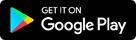Data Science Online Certification Training - DAAC## Data Science

##### Data Science

Data Science is an advanced technology that makes use of robust techniques and methodologies to analyze large volumes of Big Data. The deep data insights that are extracted from Big Data will help the enterprises to make effective business decisions. Data Science has now become a major necessity for businesses irrespective of its size. In the present age of Big Data, to stay competitive, enterprises need to efficiently develop and implement Data Science capabilities or risk being left behind.

"India has the most number of job vacancies in Data Science. This is the perfect time to step into the job market of Data Science.”

At present, the best institute for Data Course in Jaipur is DAAC. As a part of our Data Science training, participants will learn advanced technologies like Artificial Intelligence, Machine Learning, Deep Learning and TensorFlow. Advanced Data Science concepts like Business Analytics, Predictive Analytics, Forecasting, Text Mining, Hadoop and Apache Spark, Big Data Analytics are also covered as a part of this Data Science training program.

"The Learning curve in Data Science, AI & ML is steep & it opens a plethora of exciting opportunities across various industries"

### Data Science

• Duration 3 Months
• Class Timings 1:30 hr
• Eligibility Knowledge of C Language

#### MODULE - I

Introduction to Python Programming

• Introduction to Data Science
• Introduction to Python
• Basic Operations in Python
• Fundamental of Computer Graphics.
• Variable Assignment
• Creating your first Digital Painting
• Functions: in-built functions, user defined functions

#### MODULE - II

Data Structure - Introduction

• List: Different Data Types in a List
• Condition(true/false) on a List
• Applying functions on a List
• Dictionary: Index, Value
• Condition(true/false) on a Dictionary
• Applying functions on a Dictionary
• Operation on a Dictionary: Slicing, Splicing, Sub-setting
• Operations on a list: Slicing, Splicing, Sub-setting
• Loops: For, While
• Shorthand for For
• Operations on Array: Slicing, Splicing, Sub-setting
• Conditions in shorthand for For
• Conditional(T/F) on an Array

#### MODULE - III

Basics of Statistics

• Statistics & Plotting
• Seabourn & Matplotlib - Introduction
• Univariate Analysis on a Data
• Plot the Data - Histogram plot
• Find the distribution
• Find mean, median and mode of the Data
• Take multiple data with same mean but different sd, same mean and sd
• different kurtosis: find mean, sd, plot
• Multiple data with different distributions
• Bootstrapping and sub-setting
• Making samples from the Data
• Making stratified samples - covered in bi-variate analysis
• Find the mean of sample
• Central limit theorem
• Plotting
• Hypothesis testing + DOE
• Bi-variate analysis
• Correlation
• Scatter plots
• Making stratified samples
• Categorical variables
• Class variable

#### MODULE - IV

Use of Pandas

• File I/O
• Series: Data Types in series, Index
• Data Frame
• Series to Data Frame
• Re-indexing
• Pandas
• Stat operations on Data Frame
• Missing data treatment
• Merge, join
• Options for look and feel of data frame
• Writing to file
• Operations on Data Frame: Slicing, Splicing (also Alternate), Sub-setting

#### MODULE - V

Data Manipulation & Visualization

• Data Aggregation, Filtering and Transforming
• Lamda Functions
• Apply, Group-by
• Map, Filter and Reduce
• Visualization
• as
• Matplotlib, pyplot
• Seaborn
• Scatter plot, histogram, density, heat-map, bar charts

#### MODULE - VI

Linear Regression

• Regression - Introduction
• Linear Regression: Lasso, Ridge
• Variable Selection
• Forward & Backward Regression

#### MODULE - VII

Logistic Regression

• Logistic Regression: Lasso, Ridge
• Naive Bayes

#### MODULE - VIII

Unsupervised Learning

• Unsupervised Learning - Introduction
• Distance Concepts
• Classification
• Clustering
• Multidimensional Scaling
• PCA

#### MODULE - IX

Random Forest

• Decision trees
• Cart C4.5
• Random Forest
• Boosted Trees